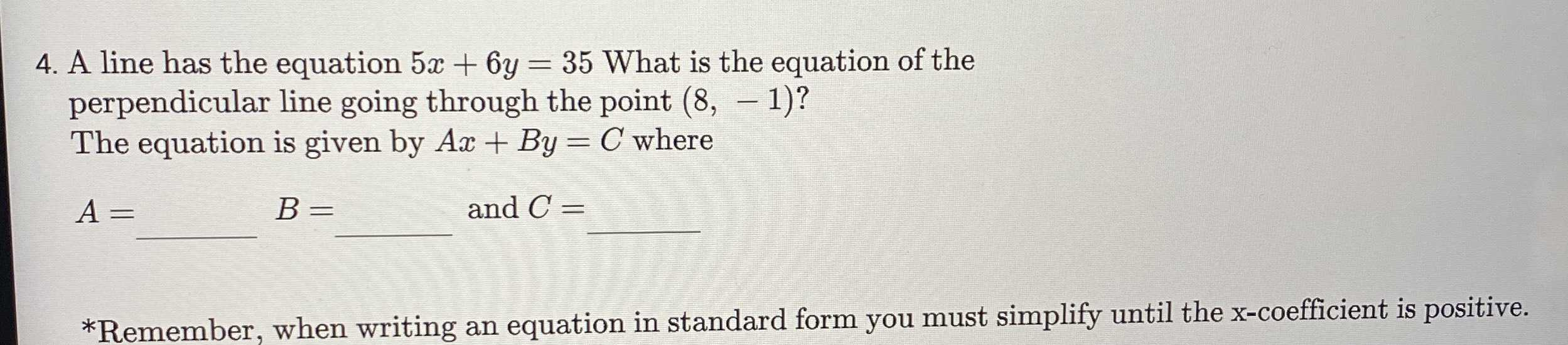### ¿Todavía tienes preguntas de matemáticas?

Pregunte a nuestros tutores expertos
Algebra
Pregunta4. A line has the equation $$5 x + 6 y = 35$$ What is the equation of the perpendicular line going through the point $$( 8 , - 1 ) ?$$ The equation is given by $$A x + B y = C$$ where $$A = \square$$ and $$C =$$

$$*$$ Remember, when writing an equation in standard form you must simplify until the x-coefficient is positive.